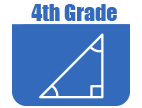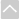OER Curriculum

These Open Educational Resources are comprehensive and coherent curricular materials that may be used to teach a course or grade level.

Engage NY
• Grade 4 Mathematics Module 1: Place Value, Rounding, and Algorithms for Addition and Subtraction
In this 25-day module of Grade 4, students extend their work with whole numbers. They begin with large numbers using familiar units (hundreds and thousands) and develop their understanding of millions by building knowledge of the pattern of times ten in the base ten system on the place value chart (4.NBT.1). They recognize that each sequence of three digits is read as hundreds, tens, and ones followed by the naming of the corresponding base thousand unit (thousand, million, billion).
• Grade 4 Mathematics Module 2: Unit Conversions and Problem Solving with Metric Measurement
Module 2 uses length, mass and capacity in the metric system to convert between units using place value knowledge. Students recognize patterns of converting units on the place value chart, just as 1000 grams is equal 1 kilogram, 1000 ones is equal to 1 thousand. Conversions are recorded in two-column tables and number lines, and are applied in single- and multi-step word problems solved by the addition and subtraction algorithm or a special strategy. Mixed unit practice prepares students for multi-digit operations and manipulating fractional units in future modules.
• Grade 4 Mathematics Module 3: Multi-Digit Multiplication and Division
In this 43-day module, students use place value understanding and visual representations to solve multiplication and division problems with multi-digit numbers. As a key area of focus for Grade 4, this module moves slowly but comprehensively to develop students' ability to reason about the methods and models chosen to solve problems with multi-digit factors and dividends.
• Grade 4 Mathematics Module 4: Angle Measure and Plane Figures
This 20-day module introduces points, lines, line segments, rays, and angles, as well as the relationships between them. Students construct, recognize, and define these geometric objects before using their new knowledge and understanding to classify figures and solve problems. With angle measure playing a key role in their work throughout the module, students learn how to create and measure angles, as well as create and solve equations to find unknown angle measures. In these problems, where the unknown angle is represented by a letter, students explore both measuring the unknown angle with a protractor and reasoning through the solving of an equation. Through decomposition and composition activities as well as an exploration of symmetry, students recognize specific attributes present in two-dimensional figures. They further develop their understanding of these attributes as they classify two-dimensional figures based on them.
• Grade 4 Mathematics Module 5: Fraction Equivalence, Ordering, and Operations
In this 40-day module, students build on their Grade 3 work with unit fractions as they explore fraction equivalence and extend this understanding to mixed numbers. This leads to the comparison of fractions and mixed numbers and the representation of both in a variety of models. Benchmark fractions play an important part in students ability to generalize and reason about relative fraction and mixed number sizes. Students then have the opportunity to apply what they know to be true for whole number operations to the new concepts of fraction and mixed number operations.
• Grade 4 Mathematics Module 6: Decimal Fractions
This 20-day module gives students their first opportunity to explore decimal numbers via their relationship to decimal fractions, expressing a given quantity in both fraction and decimal forms. Utilizing the understanding of fractions developed throughout Module 5, students apply the same reasoning to decimal numbers, building a solid foundation for Grade 5 work with decimal operations.
• Grade 4 Mathematics Module 7: Exploring Measurement with Multiplication
In this 20-day module, students build their competencies in measurement as they relate multiplication to the conversion of measurement units. Throughout the module, students will explore multiple strategies for solving measurement problems involving unit conversion.
•Georgia Standards of Excellence Curriculum Frameworks
• Grade 4 Unit 1: Whole Numbers, Place Value, and Rounding (Georgia Standards)
In this unit students will read numbers correctly through the millions, write numbers correctly through millions in standard form, write numbers correctly through millions in expanded form, identify the place value name for multi-digit whole numbers, identify the place value locations for multi-digit whole numbers, round multi-digit whole numbers to any place, fluently solve multi-digit addition and subtraction problems using the standard algorithm and solve multi-step problems using the four operations
• Grade 4 Unit 2: Multiplication and Division of Whole Numbers (Georgia Standards)
In this unit students will solve multi-step problems using the four operations, use estimation to solve multiplication and division problems, find factors and multiples, identify prime and composite numbers and generate patterns.
• Grade 4 Unit 3: Fraction Equivalents (Georgia Standards)
In this unit students will understand representations of simple equivalent fractions and compare fractions with different numerators and different denominators.
• Grade 4 Unit 4: Operations with Fractions (Georgia Standards)
In this unit students will identify visual and written representations of fractions, understand representations of simple equivalent fractions, understand the concept of mixed numbers with common denominators to 12, add and subtract fractions with common denominators, add and subtract mixed numbers with common denominators and convert mixed numbers to improper fractions and improper fractions to mixed fractions.
• Grade 4 Unit 5: Fractions and Decimals (Georgia Standards)
In this unit, students will express fractions with denominators of 10 and 100 as decimals, understand the relationship between decimals and the base ten system, understand decimal notation for fractions, use fractions with denominators of 10 and 100 interchangeably with decimals and express a fraction with a denominator 10 as an equivalent fraction with a denominator 100.
• Grade 4 Unit 6: Geometry (Georgia Standards)
In this unit, students will draw points, lines, line segments, rays, angles (right, acute, obtuse), and perpendicular and parallel lines, identify and classify angles and identify them in two-dimensional figures, distinguish between parallel and perpendicular lines and use them in geometric figures and identify differences and similarities among two dimensional figures based on the absence or presence of characteristics such as parallel or perpendicular lines and angles of a specified size.
• Grade 4 Unit 7: Measurement (Georgia Standards)
In this unit students will investigate what it means to measure length, weight, liquid volume, time, and angles, understand how to use standardized tools to measure length, weight, liquid volume, time, and angles, understand how different units within a system (customary and metric) are related to each other, know relative sizes of measurement units within one system of units including km, m, cm; kg, g; lb, oz; L, ml; hr, min, sec. and solve word problems involving distances, intervals of time, liquid volumes, masses of objects, and money, including problems involving simple fractions or decimals.
•The Online Core Resource pages are a collaborative project between the Utah State Board of Education and the Utah Education Network. If you would like to recommend a high quality resource, contact Trish French (Elementary) or Lindsey Henderson (Secondary). If you find inaccuracies or broken links contact resources@uen.org.